# Easy Way For Evaluating Equations & Functions In OneNote 2010

Unarguably OneNote 2010 is a brilliant notetaking application, providing loads of tools to facilitate the user in every possible way. Also for calculating different sort of equations, it provides the best and easiest way around. In OneNote you don’t need to use any other app to calculate any simple or even complex equations or formula. It gives the very best experience of calculating equation as if you are doing it on paper. It supports loads of functions and can evaluate many different equation. But in this post we will be focusing on evaluating basic arithmetic equations, trigonometric functions, constants etc.

Launch OneNote 2010 and move to the desired notesheet on which you want to formulate different calculations.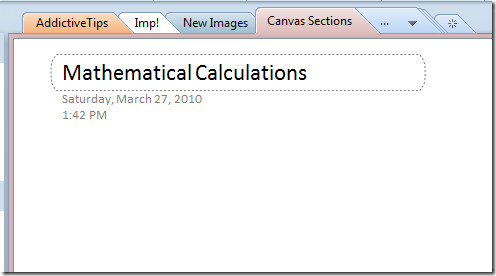### Arithmetic Equations

Enter a simple arithmetic equation to evaluate it. Just add an equal to sign at the end of the equation.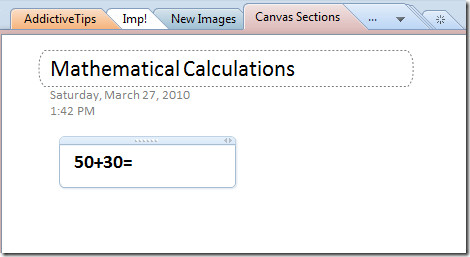Now press Space bar on keyboard, it will instantly evaluate the calculation.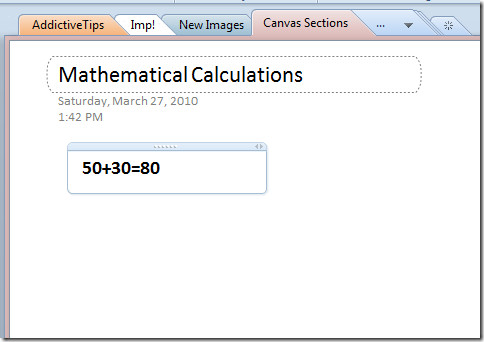You can also calculate the arithmetic equation which need to comply with operator precedent standards. OneNote can also evaluate the equation containing big numbers and complex calculations.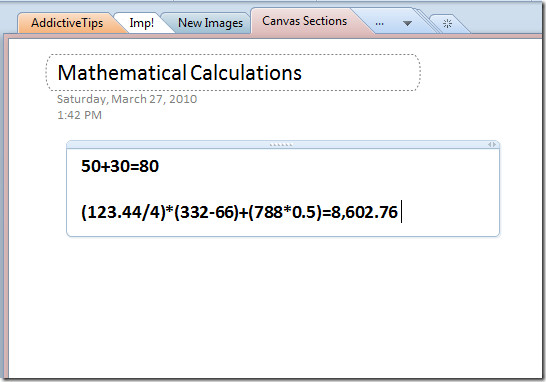Apart from evaluating simple arithmetic equations, you can go for the complex ones;

Square root: sqrt(5)=

Modulus: 32mod3=

Factorial= 9!=

Percentage= 89*20%=

Power: 3^2=

### Evaluating Functions:

OneNote has an ability to evaluate functions, which can be complex for manual formulation. For instance if you want to evaluate payment function (monthly payment on the loan), then simply write it as;

pmt(interest;numbers of payment; debt amount)

As shown in the screenshot below, it will instantly evaluate the result.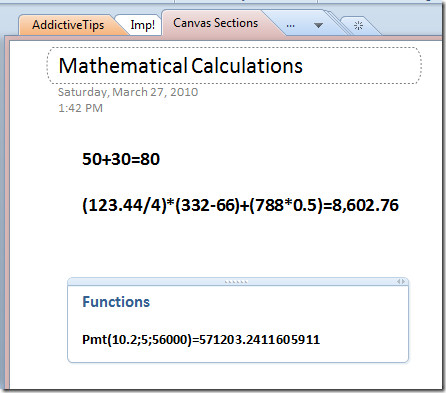You can also try out other functions in your notesheet, such as, DEG, RAD, SQRT, LOG, ABS, etc by putting in required arguments.

### Trigonometric Functions

Finding out trigonometric value could be very tedious when dealing with complex data values. OneNote 2010 instantly returns the result of trigonometric functions. For evaluating them you have got enter the degrees in small brackets followed by equal to sign.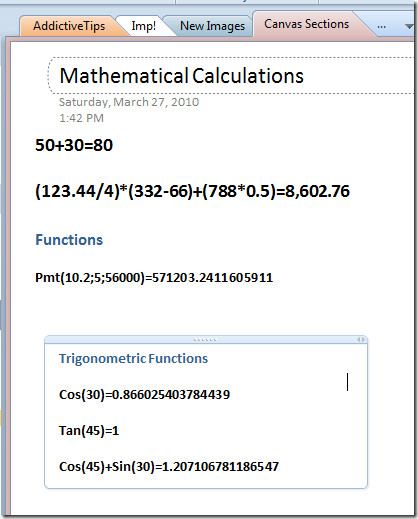### Using Constants

Now while taking notes you don’t need to remember the values of CONSTANTS, OneNote 2010 would calculate it for you, you can also punch-in arithmetic equations with it (if required).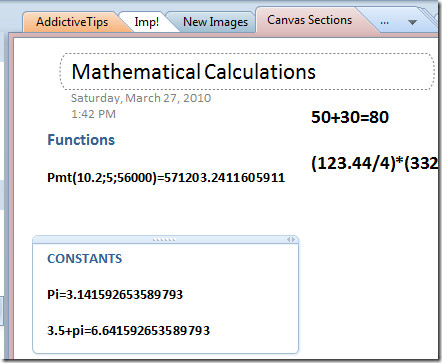You can check out previously reviewed guides on Writing mathematical Equation with hand-writing tool in OneNote & How to make notesheet password-protected.

This site uses Akismet to reduce spam. Learn how your comment data is processed.